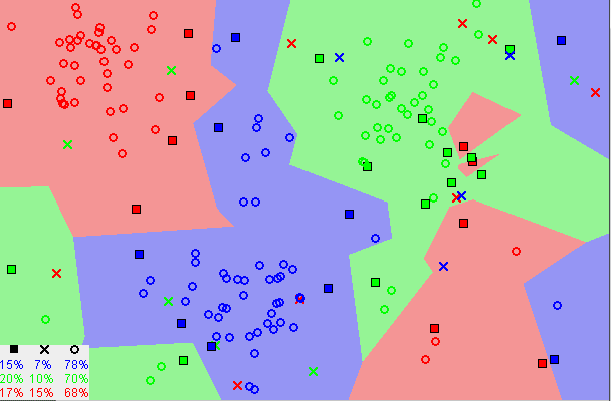# The Roadmap to Model Development Continued

Last week we took a look at the first two major steps in the process of model development, Data Preprocessing and Exploratory Data Analysis. In today’s article we will continue on the same roadmap, looking at two more steps that are arguably more interesting. These include the final data preprocessing to prep for model development, and developing the first few models.

Data Preprocessing for Prediction

What is the difference between the first data preprocessing step and the second? In the first step, you will remember that all we did was drop unwanted features. This is solely to prepare the data so we can perform Exploratory Data Analysis. In the second preprocessing step, we do all the other necessary actions for model deployment.

1. Encoding categorical features

Label encoding refers to converting the labels into a numeric form so as to convert them into the machine-readable form. We will use it on our training data, and using the “.fit” sci-kit method it will figure out the unique values and assign a value to it, returning the encoded labels.

This is what the code looks like:

1. Splitting Test and Training Data

Every machine learning model needs test and training data to learn and improve. In this step, we separate the test and training data from the dataset consisting of ten thousand values. We use the “train_test_split” module from sci-kit and “.drop” from the pandas library to get four datasets, x_train, y_train, x_test, and y_test.

This is what the code looks like:

1. Feature Scaling

Feature Scaling is a technique to normalize/standardize the independent features present in the data in a fixed range. It is done to handle highly varying magnitudes or values/units. Without feature scaling, a machine learning algorithm will weigh greater values higher and almost disregard smaller values, not taking into account the units. Note that we only do this to the x datasets, because the output does not need to be normalized.

This is what the code looks like:

Here is an image that shows how the distribution of the data is changed after feature scaling.

Model Development

One of the final and most important in any model’s development, the actual creation of the model itself. For the best accuracy possible, we will be creating multiple models and comparing their accuracy to choose the best one. In this week’s post we will look at a couple and next week we will look at the rest. We will first create a place to store our model’s names and their actual instances.

Here is the code I used to do this:

• The Logistic Regression Model

The Logistic Regression model is a supervised learning algorithm that investigates the relationship between a dependent and independent variable and produces results in a binary format (fail or not fail). This is used to predict failure.

Here is the code for the linear regression model, and for appending it to our arrays.

• K- Nearest Neighbors Model

K-Nearest Neighbors is also a supervised machine learning algorithm which is mostly used to solve classification problems. It stores all the available cases and classifies new cases based on similarity. “K” is the number of nearest neighbors.

This image shows how k-nearest neighbors uses the location of data distribution to predict.This is the code to implement the KNN model and append it:

In the next week’s blog post, we will finish the final step by creating more models, including Support Vector Machine, Random Forest, Naive Bayes, and Decision Trees. We will then test the accuracy of all of them to choose the most accurate one.